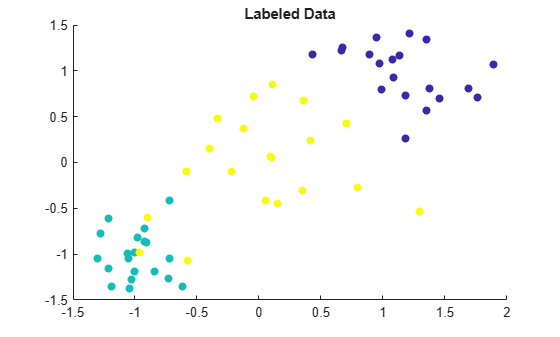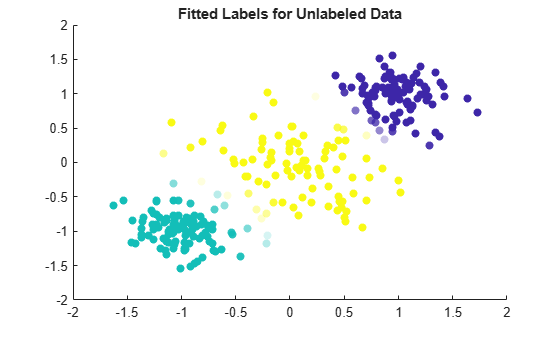# SemiSupervisedSelfTrainingModel

Semi-supervised self-trained model for classification

Since R2020b

## Description

You can use a semi-supervised self-training method to label unlabeled data by using the `fitsemiself` function. The resulting `SemiSupervisedSelfTrainingModel` object contains the fitted labels for the unlabeled observations (`FittedLabels`) and their scores (`LabelScores`). You can also use the `SemiSupervisedSelfTrainingModel` object as a classifier, trained on both the labeled and unlabeled data, to classify new data by using the `predict` function.

## Creation

Create a `SemiSupervisedSelfTrainingModel` object by using `fitsemiself`.

## Properties

expand all

Labels fitted to the unlabeled data, specified as a categorical or character array, logical or numeric vector, or cell array of character vectors. `FittedLabels` has the same data type as the class labels in the response variable in the call to `fitsemiself`. (The software treats string arrays as cell arrays of character vectors.)

Each row of `FittedLabels` represents the fitted label of the corresponding observation of `UnlabeledX` or `UnlabeledTbl`.

Data Types: `single` | `double` | `logical` | `char` | `cell` | `categorical`

Scores for the fitted labels, specified as a numeric matrix. `LabelScores` has size u-by-K, where u is the number of observations in the unlabeled data and K is the number of classes in `ClassNames`.

`score(u,k)` is the likelihood that the observation `u` belongs to class `k`, where a higher score value indicates a higher likelihood. The range of score values depends on the underlying classifier `Learner`.

Data Types: `single` | `double`

Underlying classifier, specified as a classification model object. `fitsemiself` uses this classifier in a loop to label and score the unlabeled data. You can use dot notation to display the parameter and hyperparameter values of the underlying classifier.

For example, if you specify `'Learner','svm'` in the call to `fitsemiself`, then you can enter `Mdl.Learner.KernelParameters` to display the kernel parameters of the final support vector machine (SVM) model trained on both the labeled and unlabeled data.

Note

Because the `Mdl.Learner` model has some limitations (for example, lack of support for tabular data), avoid using it directly with its object functions, such as `loss` and `predict`. To predict on new data, use the `predict` object function of `SemiSupervisedSelfTrainingModel`.

Categorical predictor indices, specified as a positive integer vector. Assuming that the predictor data contains observations in rows, `CategoricalPredictors` contains index values corresponding to the columns of the predictor data that contain categorical predictors. If none of the predictors are categorical, then this property is empty (`[]`).

Data Types: `double`

Unique class labels used to label the unlabeled data, specified as a categorical or character array, logical or numeric vector, or cell array of character vectors. The order of the elements of `ClassNames` determines the order of the classes.

Data Types: `single` | `double` | `logical` | `char` | `cell` | `categorical`

Predictor variable names, specified as a cell array of character vectors. The order of the elements of `PredictorNames` corresponds to the order in which the predictor names appear in the predictor data.

Data Types: `cell`

Response variable name, specified as a character vector.

Data Types: `char`

## Object Functions

 `predict` Label new data using semi-supervised self-trained classifier

## Examples

collapse all

Fit labels to unlabeled data by using a semi-supervised self-training method.

Randomly generate 60 observations of labeled data, with 20 observations in each of three classes.

```rng('default') % For reproducibility labeledX = [randn(20,2)*0.25 + ones(20,2); randn(20,2)*0.25 - ones(20,2); randn(20,2)*0.5]; Y = [ones(20,1); ones(20,1)*2; ones(20,1)*3];```

Visualize the labeled data by using a scatter plot. Observations in the same class have the same color. Notice that the data is split into three clusters with very little overlap.

```scatter(labeledX(:,1),labeledX(:,2),[],Y,'filled') title('Labeled Data')```Randomly generate 300 additional observations of unlabeled data, with 100 observations per class. For the purposes of validation, keep track of the true labels for the unlabeled data.

```unlabeledX = [randn(100,2)*0.25 + ones(100,2); randn(100,2)*0.25 - ones(100,2); randn(100,2)*0.5]; trueLabels = [ones(100,1); ones(100,1)*2; ones(100,1)*3];```

Fit labels to the unlabeled data by using a semi-supervised self-training method. The function `fitsemiself` returns a `SemiSupervisedSelfTrainingModel` object whose `FittedLabels` property contains the fitted labels for the unlabeled data and whose `LabelScores` property contains the associated label scores.

`Mdl = fitsemiself(labeledX,Y,unlabeledX)`
```Mdl = SemiSupervisedSelfTrainingModel with properties: FittedLabels: [300x1 double] LabelScores: [300x3 double] ClassNames: [1 2 3] ResponseName: 'Y' CategoricalPredictors: [] Learner: [1x1 classreg.learning.classif.CompactClassificationECOC] Properties, Methods ```

Visualize the fitted label results by using a scatter plot. Use the fitted labels to set the color of the observations, and use the maximum label scores to set the transparency of the observations. Observations with less transparency are labeled with greater confidence. Notice that observations that lie closer to the cluster boundaries are labeled with more uncertainty.

```maxLabelScores = max(Mdl.LabelScores,[],2); rescaledScores = rescale(maxLabelScores,0.05,0.95); scatter(unlabeledX(:,1),unlabeledX(:,2),[],Mdl.FittedLabels,'filled', ... 'MarkerFaceAlpha','flat','AlphaData',rescaledScores); title('Fitted Labels for Unlabeled Data')```Determine the accuracy of the labeling by using the true labels for the unlabeled data.

`numWrongLabels = sum(trueLabels ~= Mdl.FittedLabels)`
```numWrongLabels = 7 ```

Only 8 of the 300 observations in `unlabeledX` are mislabeled.

Use both labeled and unlabeled data to train a `SemiSupervisedSelfTrainingModel` object. Label new data using the trained model.

Randomly generate 15 observations of labeled data, with 5 observations in each of three classes.

```rng('default') % For reproducibility labeledX = [randn(5,2)*0.25 + ones(5,2); randn(5,2)*0.25 - ones(5,2); randn(5,2)*0.5]; Y = [ones(5,1); ones(5,1)*2; ones(5,1)*3];```

Randomly generate 300 additional observations of unlabeled data, with 100 observations per class.

```unlabeledX = [randn(100,2)*0.25 + ones(100,2); randn(100,2)*0.25 - ones(100,2); randn(100,2)*0.5];```

Fit labels to the unlabeled data by using a semi-supervised self-training method. The function `fitsemiself` returns a `SemiSupervisedSelfTrainingModel` object whose `FittedLabels` property contains the fitted labels for the unlabeled data and whose `LabelScores` property contains the associated label scores.

`Mdl = fitsemiself(labeledX,Y,unlabeledX)`
```Mdl = SemiSupervisedSelfTrainingModel with properties: FittedLabels: [300x1 double] LabelScores: [300x3 double] ClassNames: [1 2 3] ResponseName: 'Y' CategoricalPredictors: [] Learner: [1x1 classreg.learning.classif.CompactClassificationECOC] Properties, Methods ```

Randomly generate 150 observations of new data, with 50 observations per class. For the purposes of validation, keep track of the true labels for the new data.

```newX = [randn(50,2)*0.25 + ones(50,2); randn(50,2)*0.25 - ones(50,2); randn(50,2)*0.5]; trueLabels = [ones(50,1); ones(50,1)*2; ones(50,1)*3];```

Predict the labels for the new data by using the `predict` function of the `SemiSupervisedSelfTrainingModel` object. Compare the true labels to the predicted labels by using a confusion matrix.

```predictedLabels = predict(Mdl,newX); confusionchart(trueLabels,predictedLabels)```Only 8 of the 150 observations in `newX` are mislabeled.

## Version History

Introduced in R2020b